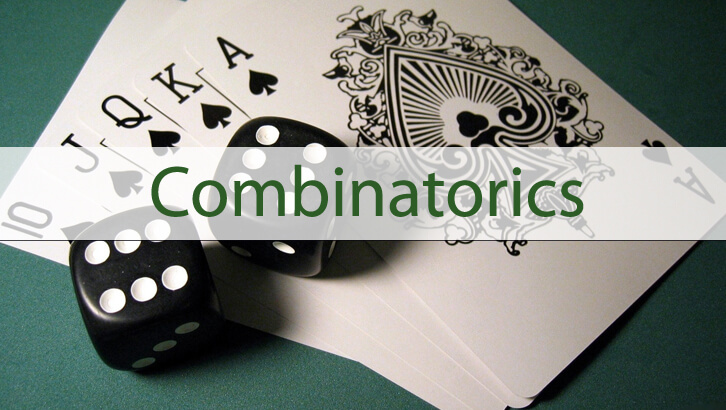# Solutions to questions on permutations and combinations

Have given below solutions to the questions on permutations and combinations

1. a, b, c are three distinct integers from 2 to 10 (both inclusive). Exactly one of ab, bc and ca is odd. abc is a multiple of 4. The arithmetic mean of a and b is an integer and so is the arithmetic mean of a, b and c. How many such triplets are possible (unordered triplets)

Exactly one of ab, bc and ca is odd => Two are odd and one is even

abc is a multiple of 4 => the even number is a multiple of 4

The arithmetic mean of a and b is an integer => a and b are odd

and so is the arithmetic mean of a, b and c. => a+ b + c is a multiple of 3

c can be 4 or 8.

c = 4; a, b can be 3, 5 or 5, 9

c = 8; a, b can be 3, 7 or 7, 9

Four triplets are possible

2. A seven-digit number comprises of only 2’s and 3’s. How many of these are multiples of 12?

Number should be a multiple of 3 and 4. So, the sum of the digits should be a multiple of 3. WE can either have all seven digits as 3, or have three 2’s and four 3’s, or six 2’s and a 3. (The number of 2’s should be a multiple of 3).

For the number to be a multiple of 4, the last 2 digits should be 32. Now, let us combine these two.

All seven 3’s – No possibility

Three 2’s and four 3’s – The first 5 digits should have two 2’s and three 3’s in some order. No of possibilities = 5!/3!*2! = 10

Six 2’s and one 3 – The first 5 digits should all be 2’s. So, there is only one number 2222232

3. How many factors of 2^5 * 3^6 * 5^2 are perfect squares?

Any factor of this number should be of the form 2^a * 3^b * 5^c. For the factor to be a perfect square a,b,c have to be even. a can take values 0, 2, 4. b can take values 0,2, 4, 6 and c can take values 0,2. Total number of perfect squares = 3 * 4 * 2 = 24

4. How many factors of 2^4 * 5^3 * 7^4 are odd numbers?

Any factor of this number should be of the form 2^a * 3^b * 5^c. For the factor to be an odd number, a should be 0. b can take values 0,1, 2,3, and c can take values 0, 1, 2,3, 4. Total number of odd factors = 4 * 5 = 20

5. A number when divided by 18 leaves a remainder 7. The same number when divided by 12 leaves a remainder n. How many values can n take?

Number can be 7, 25, 43, 61, 79.

Remainders when divided by 12 are 7 and 1.

n can take exactly 2 values

## One comment

1.kipkirui Langat says:

i have not understood clearly